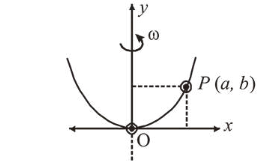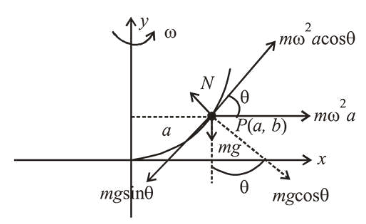# A bead of mass $m$ stays at point P(a, b)Question:

A bead of mass $m$ stays at point $P(a, b)$ on a wire bent in the shape of a parabola $y=4 C x^{2}$ and rotating with angular speed $\omega$ (see figure). The value of $\omega$ is (neglect friction) :1. (1) $2 \sqrt{2 g C}$

2. (2) $2 \sqrt{g C}$

3. (3) $\sqrt{\frac{2 g C}{a b}}$

4. (4) $\sqrt{\frac{2 g}{C}}$

Correct Option: 1

Solution:

(1) $y=4 C x^{2} \Rightarrow \frac{d y}{d x}=\tan \theta=8 C x$

At $P, \tan \theta=8 \mathrm{Ca}$$m \omega^{2} a \cos \theta=m g \sin \theta$
$\Rightarrow \omega=\sqrt{\frac{g \tan \theta}{a}}$
$\therefore \omega=\sqrt{\frac{g \times 8 a C}{a}}=2 \sqrt{2 g C}$